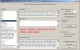TSOpenFiles 1.0 TSOpenFiles is a plugin for the REALbasic development environment. FreewareMemoryBlock 1.9 MemoryBlock is a REALbasic extension that adds the following methods and properties to the built-in MemoryBlock class: • Address (offset as Integer) as Integer • FSSpec (offset as Integer) as FolderItem (Mac OS only) • OSType (offset as Integer) as String • Int64Str (offset as Integer) as String • ULong(offset as Integer) as Double • BytesEqual(srcOfs as Integer, numBytes as Integer, destBlk as MemoryBlock, destOfs as Integer) as Boolean • FindString(srcOfs as Integer, maxBytes as Integer, target as String) as Integer • FindBytes(srcOfs as Integer, maxBytes as Integer, target as MemoryBlock, targOfs as Integer, targLen as Integer) as Integer • GetString (offset as Integer, length as Integer) as String • SetString (str as String, offset as Integer) • CopyBytes (srcOfs as Integer, numBytes as Integer, destOfs as Integer) • CopyBytes (srcOfs as Integer, numBytes as Integer, destBlk as MemoryBlock, destOfs as Integer) • CopyBytesToMacPtr (srcOfs as Integer, numBytes as Integer, destPtr as Integer) • CopyBytesFromMacPtr (srcPtr as Integer, numBytes as Integer, destOfs as Integer) • CopyBytesToMacHandle (srcOfs as Integer, numBytes as Integer, destPtr as Integer) • CopyBytesFromMacHandle (srcPtr as Integer, numBytes as Integer, destOfs as Integer). FreewareInteger Plugin 2.0 Integer Plugin for REALbasic 5 and higher allows you to construct programs which can handle unsigned 64-bit, signed 64-bit, and unsigned 32-bit integers. FreewareInteger Tiles 1.2 Integer Tiles is an education program that focuses on mental-math practice for combining integers. FreewareHIME: Huge Integer Math and Encryption 1.04 Encryption functions (public key/ secret key) and huge integer math functions. SharewareHIME: Huge Integer Math and Encryption 2.05.1 Encryption functions (public key/ secret key) and huge integer math functions SharewareBase Converter 3.2 Base Converter converts an integer expressed in one base to an expression in another base. FreewareRomanumerator 0.1 Romanumerator is a nifty program for translating between arabic and roman numeral notations. Freeware24U Hex OSAX 2.0 24U Hex OSAX is a Scripting Addition for converting text and integer numbers to hexadecimal notation and back, making life easier for programmers and web developers! The hexadecimal notation contains only alphanumeric characters, so you can utilize it for transfering data simply between platforms and over the Internet. SharewareiCalculus 1.0 iCalculus is a Programmable Graphical Calculator with the following features: • Operates with Integer, Real, Complex, Matrix and String. Shareware